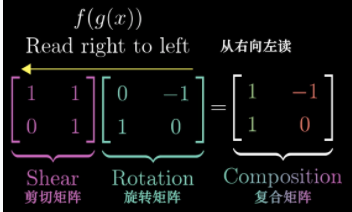## 导言

B站线代神教学地址：http://www.bilibili.com/video/av6731067

## 线性组合和矩阵、线性变换

i,j都是长度为1，成为基向量， 俩个不共线的向量通过线性组合可以张成整个空间， “线性相关”就是他可以被别人线性表达出来，一般共线（二维）或者共面（三维）的某个向量可以被其他向量线性表达出来，

• 1、原点不变
• 2原始网格按比例缩放保持直线不能弯曲。a,c就是i(1,0)变换后的坐标，b,d就是j(0,1)变换后的坐标， [x,y]就是原有的向量，[ax+by, cx+dy]就是变换后的向量 所以，矩阵本质是对一个变换的描述。## 行列式## 逆、秩、核$A$变化后，在通过$A^{-1}$变换回去，就相当于又把基坐标变回了$i,j$

“秩”表示变换后的维度，比如矩阵$A$把2维一不小心变换到了1维，那A的秩就是1，否则就是2。

## 点乘## 基变换、相似

$A^{-1} * P * A * v = v’$

• v是jeniffer的世界里的向量，
• A是把她的坐标变换成我们的世界的坐标，
• P是在我们的世界做变换，

$A^{-1}$是把我们的世界变换回Jeniffer的世界，

（这里，把A不看做是一个变换，而是一个另一个变化后的空间的基的坐标，所谓你的世界）

## 特征向量

$AV=\lambda*V$

$AV-\lambda*V=0$

$(A - \lambda*I) * V = 0$

## 其他资料

### 矩阵

$A_{ij}=(-1)^(i+j) *Mij$,

$AxA*= A*xA=|A|xE$

$|A|=0$

$|A|!=0$满秩，$r(A)=n$A可逆 叫做非奇异矩阵

A的各行、各列组成的向量组线性无关 $Ax=4$，只有零解 ？？？？这个需要论证 $Ax=B$，有唯一解 A与E等价

• 矩阵相似 $P^{-1}AP = B$ ，A和B相似，相似比等价强
• 矩阵等价 $PAQ=B$ ，P、Q都是可逆的，A、B等价意味着A=>B（A经过初等变化可以得到B）表示成
• 矩阵正定 $X^T*A*X>0$ ，A为正定阵，>=0, 半正定；正定阵特征值都是正，对称阵是正定阵
• 矩阵可逆 行列式$Det(A)=0$，就是可逆的。
• 矩阵合同 $P^TAP = B$ P可逆，A和B合同， 合同比等价强，合同和相似没有任何关系（除非这个矩阵是正交矩阵），正交合同和
• 正交矩阵 $PP^T=E$ ，$P^{-1}=P^T$,正交矩阵的逆等于他的转置

### 逆矩阵

1. $A^{-1} = \frac{1}{|A|} x A^*$ 参考伴随矩阵的性质

2. 做初等变化得到 $(A|E)=>(E| A^{-1} )$, 原因是A可逆，A就是满秩的，满秩的就可以写成一系列初等矩阵的乘积（初等矩阵的性质）

### 向量&向量空间

• n维向量组成组成m行矩阵,如果矩阵秩$r(A)<m$,向量组线性相关;$r(A)=m$,向量组线性无关.(跟矩阵的线性无关判定一样)
• 向量组线性无关,向量组每人维度+1,她们之间还是线性无关.
• 向量组的等价:俩向量组彼此可以线性表示.
• 极大线性无关组的向量个数叫向量组的秩.
• 基: 指这样一个向量组,它可以表示向量空间的所有的向量,并且他们之间线性无关.
• 也就是极大线性无关组,个数就是组成矩阵的秩.
• 如何求秩\基\极大线性无关组: 是把向量按照”列!”方式码放,然后做行变化,化成梯形阵, 或者按照”行!”方式码放,然后做列变化,化成梯形阵, 向量组的任意两个极大线性无关组包含向量个数相等. 等价向量组的秩相同.

### 特征向量

$AX=lambda*X$

$\lambda$是特征值,$X$是特征向量,

$|A-\lambda*I|=0$,行列式为0,可以求出$\lambda$, 不同特征值对应的特征向量彼此线性无关,也就是彼此无法线性表出 求特征值和特征向量的方法:$|A - \lambda * E | = 0$,解出$\lambda$,然后$(A-\lambda E)X=0$,所有的X都是特征向量.

1.实对称矩阵A的不同特征值对应的特征向量是正交的。

2.实对称矩阵A的特征值都是实数，特征向量都是实向量。

3.n阶实对称矩阵A必可对角化，且相似对角阵上的元素即为矩阵本身特征值。

### 相似对角化

$Q^{-1} A Q = \Lambda$ , 其中 $\Lambda是一个对角阵，对角线上是特征是\lambda_i$。

$Q^{-1} A Q = Q^T A Q = \Lambda$

### PCA

PCA干嘛用的呢？

• 1）将原始数据按列组成n行m列矩阵X
• 2）将X的每一行（代表一个属性字段）进行零均值化，即减去这一行的均值
• 3）求出协方差矩阵
• 4）求出协方差矩阵的特征值及对应的特征向量
• 5）将特征向量按对应特征值大小从上到下按行排列成矩阵，取前k行组成矩阵P
• 6）Y=PX即为降维到k维后的数据

• 矩阵P是由C的特征向量组成的，而且是由比较大的特征值对应的向量组成的。
• 这个特征向量已经是完全和之前的特征值无关的了，之前是40000维度哈，现在呢，只是k了，这k可不是从40000里跳出来的，而是完全没关系的，只是通过特征值分解得到的了。
• 另外，特征值得出的所依赖的矩阵C，也不是原始的X，是X和他转置相乘的结果，是一个对称阵（对称阵才可以得到正定的特征值），而且X也不是原来的我，而是均值化的了，我早已不是我了
• 总而言之，整个数学变化，就是为了得到对应的特征向量

## 参考

http://www.cnblogs.com/zhanjxcom/p/4119509.html

http://blog.codinglabs.org/articles/pca-tutorial.html

SVD分解 小象学院的《课时9 视频4 矩阵和线性代数》